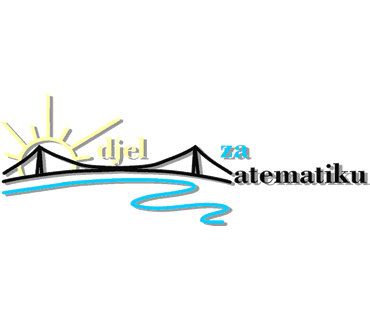## Poziv na predavanje na engleskom jeziku Accurate eigenvalue decomposition of real symmetric arrowhead matrices (…)

#### Odjel za matematiku Sveučilišta Josipa Jurja Strossmayera u Osijeku organizira predavanje Accurate eigenvalue decomposition of real symmetric arrowhead matrices and rank-one modifications of diagonal matrices and applications, na engleskom jeziku

NAJAVA – Predavanje će se održati u četvrtak, 15. svibnja 2014. godine s početkom u 17 sati u predavaonici broj 36 na Odjelu za matematiku Sveučilišta Josipa Jurja Strossmayera u Osijeku (Trg Ljudevita Gaja 6).

Predavanje će održati:

• dr. Nevena Jakovčević Stor, Faculty of Electrical Engineering, Mechanical Engineering and Naval Architecture, University of Split

S obzirom da je riječ o predavanju na engleskom jeziku, na tom jeziku objavljujemo i kratak opis predavanja:

A novel class of forward stable algorithms for solving eigenvalue problems for n × n real symmetric arrowhead matrices and rank-one modifications of diagonal matrices is resented. The algorithms compute all eigenvalues and all components of the corresponding eigenvectors with high relative accuracy in O(n) operations per eigenvalue/eigenvector. The algorithms are based on a shift-and- invert approach. Only a single element of the inverse of the respective shifted matrix eventually needs to be computed with double the working precision. Each eigenvalue and the corresponding eigenvector can be computed separately, which makes the algorithms adaptable for parallel com- puting. Our results can also be applied to Hermitian matrices, singular value decompositions, and computing accurate zeros of polynomials. The methods can be used as a part of divide-and conquer methods for tridiagonal problems.